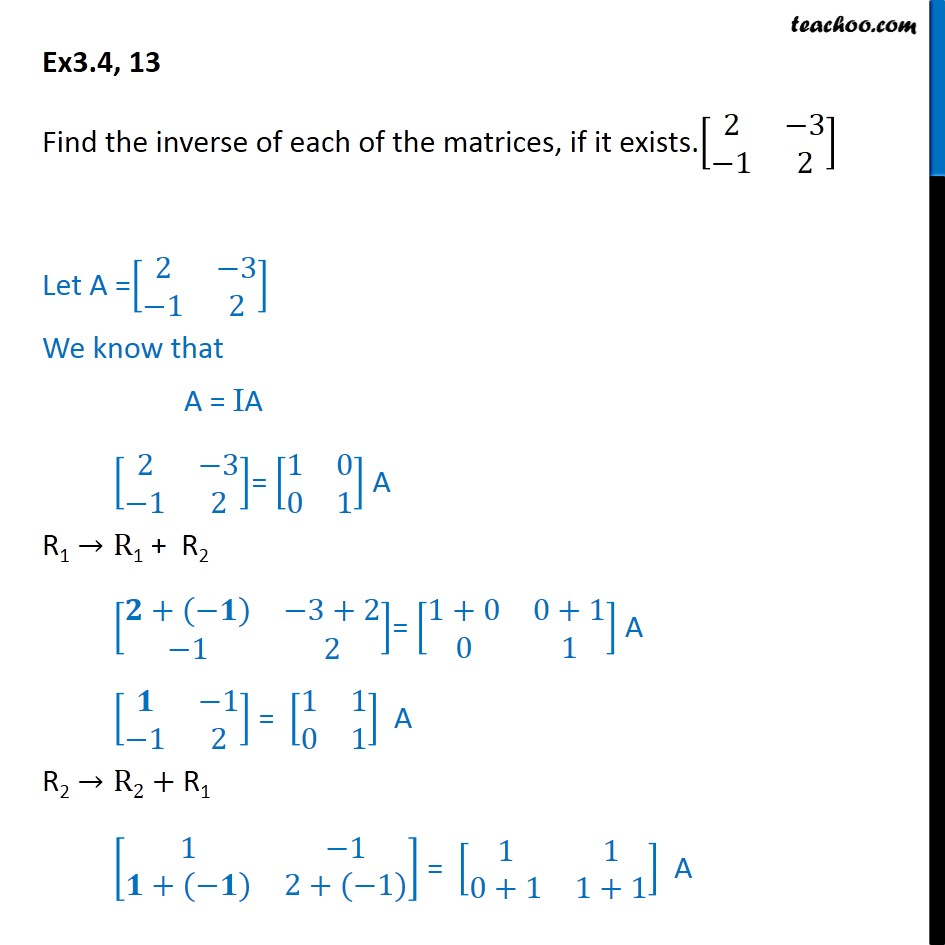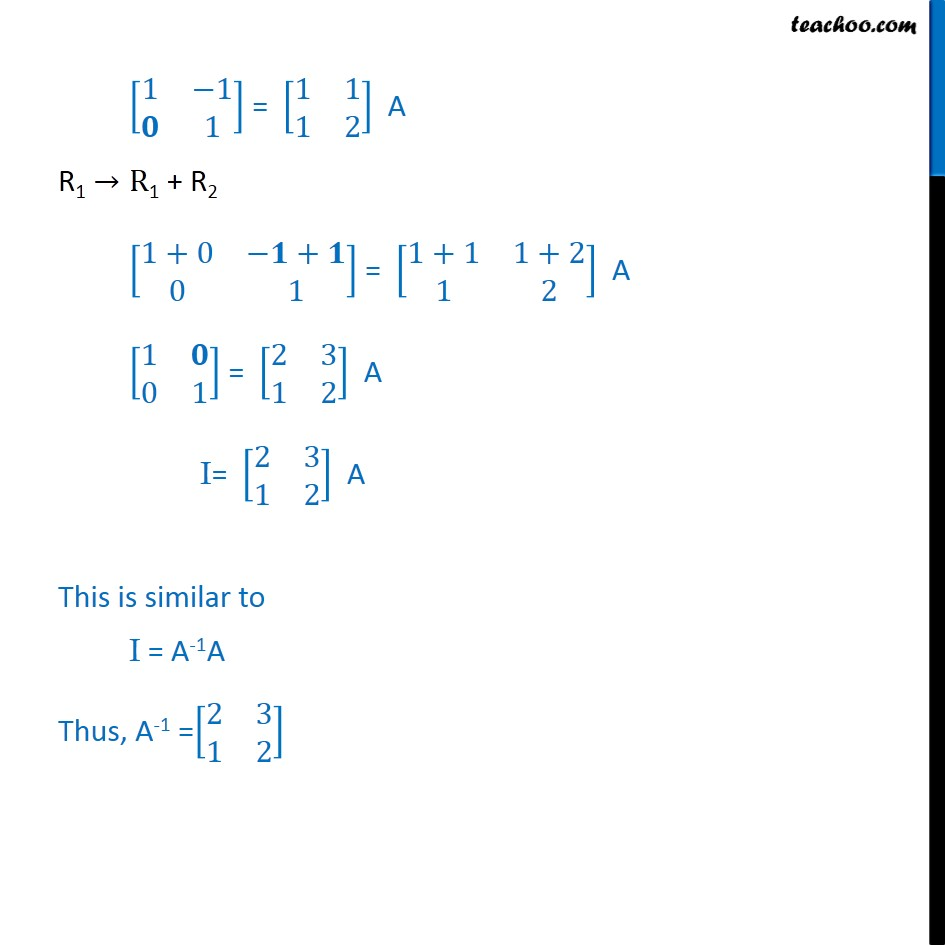1. Chapter 3 Class 12 Matrices
2. Concept wise
3. Inverse of matrix using elementary transformation

Transcript

Ex3.4, 13 Find the inverse of each of the matrices, if it exists.[ 8(2& 3@ 1&2)] Let A =[ 8(2& 3@ 1&2)] We know that A = IA [ 8(2& 3@ 1&2)]= [ 8(1&0@0&1)] A R1 R1 + R2 [ 8( +( )& 3+2@ 1&2)]= [ 8(1+0&0+1@0&1)] A [ 8( & 1@ 1&2)] = [ 8(1&1@0&1)] A R2 R2+ R1 [ 8(1& 1@ +( )&2+( 1))] = [ 8(1&1@0+1&1+1)] A [ 8(1& 1@ &1)] = [ 8(1&1@1&2)] A R1 R1 + R2 [ 8(1+0& + @0&1)] = [ 8(1+1&1+2@1&2)] A [ 8(1& @0&1)] = [ 8(2&3@1&2)] A I= [ 8(2&3@1&2)] A This is similar to I = A-1A Thus, A-1 =[ 8(2&3@1&2)]

Inverse of matrix using elementary transformation